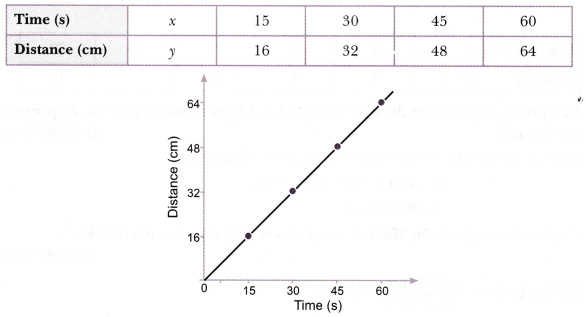# Plot a distance-time graph of the tip of the second hand of a clock by selecting 4 points on x-axis and y-axis respectively. The circumference of the circle traced by the second hand is 64 cm

Plot a distance-time graph of the tip of the second hand of a clock by selecting 4 points on x-axis and y-axis respectively. The circumference of the circle traced by the second hand is 64 cm.